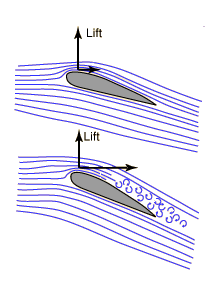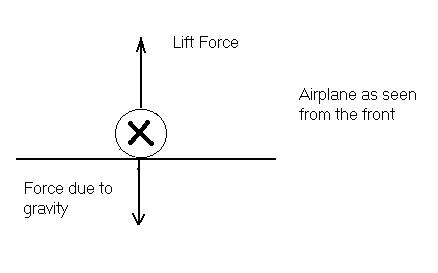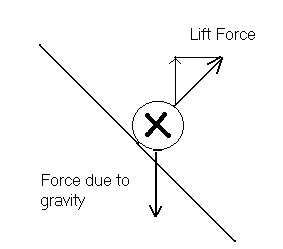The Stall Point

The stall point is where the lift force is less than the force of gravity. Here, we will investigate two ways a stall can happen.

How long can we increase the angle until the lift force no longer increases?

The lift force is generated by turning a moving fluid. This turning however will break down if the flow ceases to be laminar. Turbulence begins to happenIn this picture we can see the depiction of how turbulence increases as the angle of attack increases.

This same idea can also be seen when SUVs get dirty. Why is it that the back gets dirty? This would not happen if the air maintained laminar flow off the back of the SUV. You can see then that there is turbulent flow at the back of this automobile that picks up dirt and deposits it there.

So then there is turbulent flow that begins to occur as shown above if the angle of attack increases.

Demonstration

To show that the stall point will eventually happen, set the wing at an angle of 35° on the protractor on the cart (not on the vertical protractor). For the construction of the general apparatus see Construction of Demonstration. Attach the spring and hold the cart (spring unstretched). Turn the wind generator on and pull on the spring to make sure the cart always stays in the same place. The length that the spring stretches can be used to give an idea of relative forces as F = -kx. Note the length of the spring. Increase the angle of attack by 5° until the force begins to drop. By this I mean in crease the angle of attack until the length if the spring begins to decrease (This should occur at around 50°). This is where the lift is beginning to decrease, if this was the wing of a real airplane and we were to continue along in this way we would reach a point where the plane would stall.

The second stall point is a fairly simple extension of this it is called a banking stall. It most often occurs when a plane takes off at too steep of an angle and begins to lose lift and attempts to turn back to the airport. Pilots are warned not to do this as there is a high risk of stalling. This is why:Here we can see that the force due to gravity is almost as large as the lift force. At this point if the pilot decides he/she would like to turn back this is what would happen:We can see here that by banking the plane decreases the amount of force to resist gravity and will stall.

Demonstration

With the plane at its final angle from the previous demonstration now tilt it only along the vertical protractor by 45° and measure how the length of the spring as done previously. The lift should decrease.

Back to Introduction